KOA4
 Name:    KOA4

Multiple Choice
Identify the choice that best completes the statement or answers the question.

1.

How many more do you need to make 10?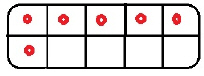a. 2 c. 3 b. 4 d. 5

2.

How many more do you need to make 10?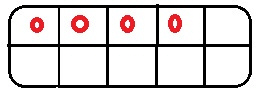a. 10 c. 3 b. 6 d. 9

3.

How many more do you need to make 10?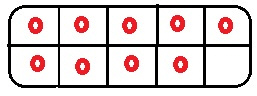a. 9 c. 4 b. 6 d. 1

4.

How many more do you need to make 10?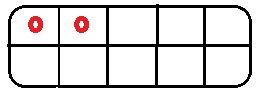a. 8 c. 3 b. 5 d. 1

5.

How many more do you need to make 10?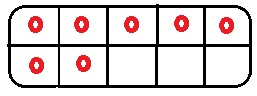a. 8 c. 3 b. 4 d. 7

6.

How many more do you need to make 10?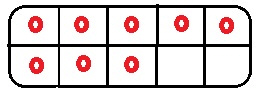a. 1 c. 9 b. 2 d. 7

7.

How many more do you need to make 10?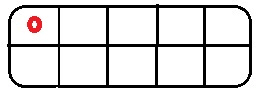a. 1 c. 9 b. 5 d. 8

8.

How many more do you need to make 10?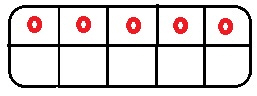a. 1 c. 3 b. 5 d. 4

9.

How many more do you need to make 10?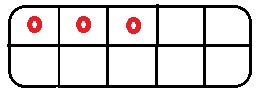a. 8 c. 7 b. 6 d. 4

10.

How many more do you need to make 10?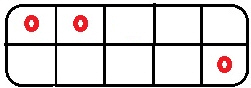a. 7 c. 9 b. 3 d. 5